# Even / odd numbers

a / Using variable n write two consecutive odd numbers.
b / the sum of three consecutive odd numbers is 333. What are this numbers?

a =  109
b =  111
c =  113

### Step-by-step explanation:

$b=a+2=111$
$c=a+4=113$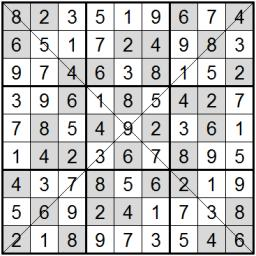Did you find an error or inaccuracy? Feel free to write us. Thank you!Tips to related online calculators
Do you have a linear equation or system of equations and looking for its solution? Or do you have a quadratic equation?

## Related math problems and questions:

• Sum of four numbers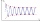The sum of four consecutive natural numbers is 114. Find them.
• RemaindersIt is given a set of numbers { 170; 244; 299; 333; 351; 391; 423; 644 }. Divide this numbers by number 66 and determine set of remainders. As result write sum of this remainders.
• Sum of sevenThe sum of seven consecutive odd natural numbers is 119. Determine the smallest of them.
• Divisible by 5How many three-digit odd numbers divisible by 5, which are in place ten's number 3?
• Even/odd numbersWhat is the product of 0.97 and the next odd decimal number?
• The sumThe sum of five consecutive odd numbers is 75. Find out the sum of the second and fourth of them.
• The sum 12The sum of 3 mixed numbers is 20 13/15. two of the numbers are 6 1/3 and 7 5/6. what is the third number?
• Dropped sheetsThree consecutive sheets dropped from the book. The sum of the numbers on the pages of the dropped sheets is 273. What number has the last page of the dropped sheets?
• QuotientFind quotient before the bracket - the largest divisor 51 a + 34 b + 68 121y-99z-33
• Odd numbersThe sum of four consecutive odd numbers is 1048. Find those numbers ...
• Decomposition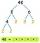Make decomposition using prime numbers of number 155. Result write as prime factors (all, even multiple)
• Expressions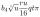Expression (3a-7).(3a+7) is identical with: ?
• Ratio three numbers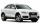Three numbers SUV are in the ratio 1:2:3. Their sum is 24. Find this numbers and write their add and sum.
• Three integersThree consecutive integers add up to 51. what are these integers?
• What are 3What are the two digits which when inserted in the blank spaces will make 234 _ _ divisible by 8?
• Two numbers 7The sum of two consecutive even numbers is 30. Find the numbers.
• Sum and roundingI know two numbers whose sum is 20. When they each round and then added together I get the 30. What are this numbers?